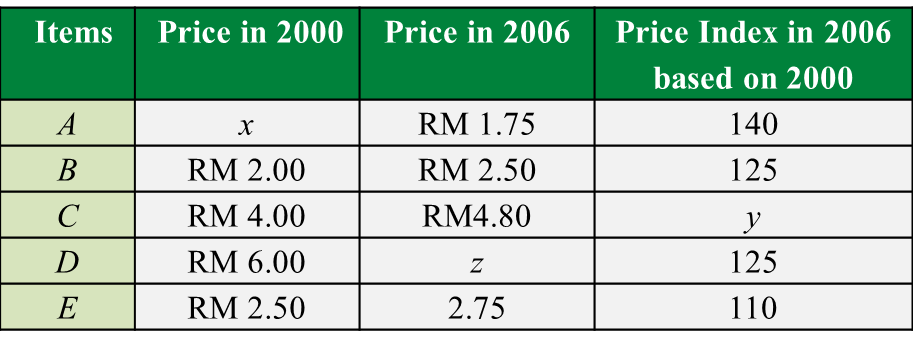\

# 10.3.3 Index Number Long Questions (Question 3)

Question 3:
Diagram below is a bar chart indicating the weekly cost of the items A, B, C, D and E  for the year 2000. Table below shows the prices and the price indices for the items.(a) Find the value of
(i) x
(ii) y
(iii) z

(b) Calculate the composite index for items in the year 2006 based on the year 2000.

(c)
The total monthly cost of the items in the year 2000 is RM456. Calculate the corresponding total monthly cost for the year 2006.

(d)
The cost of the items increases by 15 % from the year 2006 to the year 2009.
Find the composite index for the year 2009 based on the year 2000.

Solution:
(a)(i)
$\begin{array}{l}I=\frac{{P}_{1}}{{P}_{0}}×100\\ I=\frac{{P}_{2006}}{{P}_{2000}}×100\\ 140=\frac{1.75}{x}×100\\ x=\text{RM}1.25\end{array}$

(a)(ii)
$\begin{array}{l}I=\frac{{P}_{2006}}{{P}_{2000}}×100\\ y=\frac{4.80}{4.00}×100\\ y=120\end{array}$

(a)(iii)
$\begin{array}{l}I=\frac{{P}_{2006}}{{P}_{2000}}×100\\ 125=\frac{z}{6.00}×100\\ z=\text{RM7}\text{.50}\end{array}$

(b)(c)

(d)